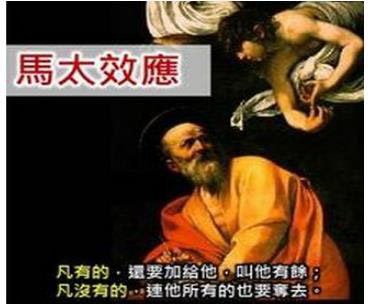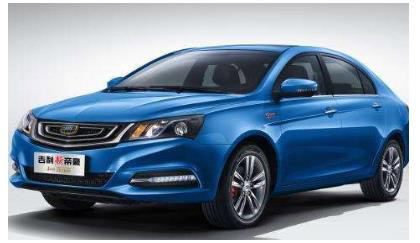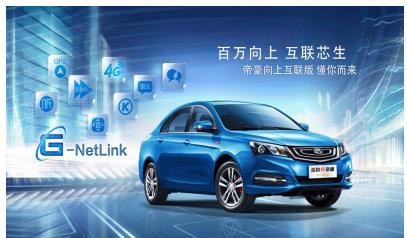## 关于NBA巨星抱团的说法 帝豪有个不成熟的小意见

2017年06月20日 10:01 来源：www.chextx.com 超过：次关注

看到金州勇士队盛大欢腾的冠军游行，勒布朗·詹姆斯心中应该会想起去年克利夫兰夺冠时同样的景象，真是“年年岁岁花相似，岁岁年年人不同”！不是小皇帝实力不强，这个进入联盟15个年头的“老汉”已经不需要再做什么来证明自己，回应那些甚嚣尘上的恶意指责。然而他确实输掉了2016-2017赛季的NBA总决赛，错失了进一步荣耀加身和提升自己历史地位的机会。相比去年两队的对决，放眼望去，很明显，勇士的阵中多了一个七尺长人——杜兰特。因为他的存在，骑士没能重演去年在1:3落后情况下上演历史大逆转的剧本，而是4:1干脆地输掉了比赛。赛后虽然詹姆斯和杜兰特都纷纷对自己效力一支“超级球队”予以否认，但事实真是这样吗？

时间回到2010年夏天，勒布朗·詹姆斯离开骑士，与德维恩·韦德、克里斯·波什组成巅峰三巨头，并在接下来完成了四年两冠两亚的成就；2014年，詹姆斯回到骑士，再度与欧文、勒夫组成三巨头，三进总决赛并于2015年夺得NBA当年的总冠军。同样的，2016年夏天，因为刚刚在总决赛折戟沉沙，已经拥有了两位历史级的射手、并创造了常规赛73胜记录的勇士，吸引到一个拥有历史罕见得分能力的联盟MVP——凯文·杜兰特的加入，从强到更强，现在这支勇士队已经所向披靡、无可阻挡。虽然詹姆斯和杜兰特的作为被很多人称之为叛徒和抱团，但无可否认的是，在他们这种优势资源强势聚合之下，NBA近些年的竞争更具看点，赛场内外也更具话题性和关注度。今年勇士和骑士的总决赛收视率破历史记录，就说明了这一点。对一个商业联盟来说，这不可谓不是一种成功。而对詹姆斯和杜兰特等球员来说，能有机会与更多伟大的球员并肩战斗，并一同斩获荣誉，甚至一起开创一个时代，无疑也是一名职业球员毕生的追求。

因此，当我们以更冷静的眼光审视NBA近年的发展，更多优秀球员开不断聚集、开始走向合作，塑造出一个个强大的“超级球队”，无论是比赛的看点和未来的期望上，都对球迷有个更大的吸引力，对于商业发展而来，“超级强队”已然成为必然的产物。说到这里，大家或许会不禁想到一个说法——马太效应。没错，让强者更强，让弱者愈弱，如今NBA的态势，已经成为这一现象的佐证。事实上，又何止是NBA，在如今各行各业，“马太效应”正大行其道：没有阿里巴巴，电子商务就不会延伸到生活的方方面面；没有滴滴，人们也很难想象出行会如此多元和便利；没有腾讯，移动社交也不会如此丰富、有趣……

从这些比比皆是的例子中，我们不难发现，强者更强的“马太效应”，为我们带来的是更集中的资源积聚和更好的服务供应。再将目光移到如今的中国汽车产业，SUV的火爆，让很多自主品牌趋之若鹜，纷纷扎堆到SUV市场。然而纵观国外汽车品牌，那些汽车强企无不适轿车、SUV等车型全面发展的典范。如今市场暂时的成功，已经让中国汽车产业发展越来越有偏科的倾向。

凡事总有例外，作为中国品牌汽车的代表，吉利汽车的发展则更为均衡，不仅SUV势头强劲，更重要的是，吉利的轿车发展更是一骑绝尘。尤其是吉利帝豪，作为吉利的销量当担，上市八年来，帝豪累计销量突破126万辆，连续6年位居中国品牌轿车销量冠军，并对此跻身轿车月销量排行前十名，成为中国品牌对标合资品牌的生力军，成为是中国品牌轿车名副其实的代表和典范。纵观吉利帝豪的发展，以只为向上、积极拼搏的品牌理念，不断完成产品的更新升级，塑造中国轿车市场“强者更强”的传奇佳话，帝豪已然成为汽车界“马太效应”的典型案例。

上市八年，帝豪历经七次改款升级，每一次都成功切中时代趋势、抓住用户痛点，完成品质和实力的全面升级。2016年，纪念百万销量的新帝豪百万款发布，在造型、安全、舒适及性能功能等方面多达33项优化升级，为下一个百万销量注入了强劲的动力；立足移动互联的时代基调和智能互联的用户需求，近期，帝豪实力推出新帝豪百万款向上互联版，以硬件、网络、导航和智能娱乐等方面的性能提升，为用户带来“快、准、全、趣”的智能互联体验。资源优势集结之下实现的自我实力愈来愈强，詹姆斯和杜兰特用实际行动为大家展现了“超级球队”是如何成就自己职业生涯的辉煌成就的。而在中国品牌轿车领域，吉利帝豪以同样的方式，在不断的自我更新和升级中，演绎了中国汽车领域的“马太效应”，捍卫了中国品牌轿车的荣耀。以“强者更强”的姿态，帝豪当之无愧地成为了“更懂国人的车”。

#### 相关文章

﻿
• 快速找车
• 选择品牌
• 选择品牌
• A  奥迪
• A  阿斯顿·马丁
• A  阿尔法·罗密欧
• B  宝沃
• B  布加迪
• B  巴博斯
• B  保时捷
• B  宾利
• B  奔驰
• B  宝马
• B  本田
• B  别克
• B  标致
• B  比亚迪
• B  宝骏
• B  北汽制造
• B  北汽新能源
• B  北汽幻速
• B  北汽威旺
• B  北京汽车
• B  奔腾
• B  北汽绅宝
• B  北汽昌河
• C  长安欧尚
• C  长安
• C  长安凯程
• C  长城
• D  大众
• D  道奇
• D  DS
• D  东南
• D  东风风神
• D  东风风行
• D  东风小康
• D  东风风度
• D  东风
• F  福特
• F  丰田
• F  菲亚特
• F  法拉利
• F  福田
• F  福迪
• F  福汽启腾
• G  观致
• G  广汽传祺
• G  广汽吉奥
• G  GMC
• H  红旗
• H  汉腾汽车
• H  哈弗
• H  哈飞
• H  海格
• H  海马
• H  华颂
• H  黄海
• H  华泰
• H  恒天
• J  捷途
• J  几何汽车
• J  捷达
• J  吉利汽车
• J  捷豹
• J  Jeep
• J  江淮
• J  江铃
• J  金杯
• J  九龙
• J  金旅
• K  凯翼
• K  凯迪拉克
• K  克莱斯勒
• K  科尼塞克
• K  卡威
• K  开瑞
• L  路虎
• L  林肯
• L  劳斯莱斯
• L  兰博基尼
• L  雷克萨斯
• L  铃木
• L  领克
• L  雷诺
• L  理念
• L  力帆
• L  莲花汽车
• L  猎豹
• L  路特斯
• L  陆风
• M  马自达
• M  MG
• M  MINI
• M  玛莎拉蒂
• M  摩根
• M  迈凯轮
• N  纳智捷
• O  欧拉
• O  欧宝
• O  讴歌
• O  欧朗
• Q  奇瑞
• Q  起亚
• Q  启辰
• R  日产
• R  荣威
• R  瑞麒
• S  SERES赛力斯
• S  三菱
• S  斯威汽车
• S  萨博
• S  smart
• S  斯柯达
• S  斯巴鲁
• S  思铭
• S  双龙
• S  上汽大通
• S  双环
• T  特斯拉
• T  腾势
• W  蔚来
• W  沃尔沃
• W  WEY
• W  五菱汽车
• W  五十铃
• W  威兹曼
• W  威麟
• X  现代
• X  雪佛兰
• X  星途
• X  雪铁龙
• X  小鹏汽车
• X  西雅特
• Y  一汽
• Y  英菲尼迪
• Y  英致
• Y  依维柯
• Y  野马汽车
• Y  永源
• Z  众泰
• Z  中华
• Z  中兴
• Z  知豆
• 选择车系
• 选择车系
• 车型对比
• 选择品牌
• 选择品牌
• A  奥迪
• A  阿斯顿·马丁
• A  阿尔法·罗密欧
• B  宝沃
• B  布加迪
• B  巴博斯
• B  保时捷
• B  宾利
• B  奔驰
• B  宝马
• B  本田
• B  别克
• B  标致
• B  比亚迪
• B  宝骏
• B  北汽制造
• B  北汽新能源
• B  北汽幻速
• B  北汽威旺
• B  北京汽车
• B  奔腾
• B  北汽绅宝
• B  北汽昌河
• C  长安欧尚
• C  长安
• C  长安凯程
• C  长城
• D  大众
• D  道奇
• D  DS
• D  东南
• D  东风风神
• D  东风风行
• D  东风小康
• D  东风风度
• D  东风
• F  福特
• F  丰田
• F  菲亚特
• F  法拉利
• F  福田
• F  福迪
• F  福汽启腾
• G  观致
• G  广汽传祺
• G  广汽吉奥
• G  GMC
• H  红旗
• H  汉腾汽车
• H  哈弗
• H  哈飞
• H  海格
• H  海马
• H  华颂
• H  黄海
• H  华泰
• H  恒天
• J  捷途
• J  几何汽车
• J  捷达
• J  吉利汽车
• J  捷豹
• J  Jeep
• J  江淮
• J  江铃
• J  金杯
• J  九龙
• J  金旅
• K  凯翼
• K  凯迪拉克
• K  克莱斯勒
• K  科尼塞克
• K  卡威
• K  开瑞
• L  路虎
• L  林肯
• L  劳斯莱斯
• L  兰博基尼
• L  雷克萨斯
• L  铃木
• L  领克
• L  雷诺
• L  理念
• L  力帆
• L  莲花汽车
• L  猎豹
• L  路特斯
• L  陆风
• M  马自达
• M  MG
• M  MINI
• M  玛莎拉蒂
• M  摩根
• M  迈凯轮
• N  纳智捷
• O  欧拉
• O  欧宝
• O  讴歌
• O  欧朗
• Q  奇瑞
• Q  起亚
• Q  启辰
• R  日产
• R  荣威
• R  瑞麒
• S  SERES赛力斯
• S  三菱
• S  斯威汽车
• S  萨博
• S  smart
• S  斯柯达
• S  斯巴鲁
• S  思铭
• S  双龙
• S  上汽大通
• S  双环
• T  特斯拉
• T  腾势
• W  蔚来
• W  沃尔沃
• W  WEY
• W  五菱汽车
• W  五十铃
• W  威兹曼
• W  威麟
• X  现代
• X  雪佛兰
• X  星途
• X  雪铁龙
• X  小鹏汽车
• X  西雅特
• Y  一汽
• Y  英菲尼迪
• Y  英致
• Y  依维柯
• Y  野马汽车
• Y  永源
• Z  众泰
• Z  中华
• Z  中兴
• Z  知豆
• 选择车系
• 选择车系
• 选择车型
• 选择车型
• 意见反馈